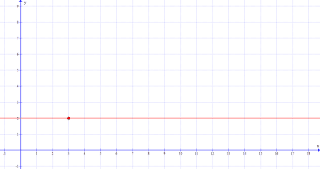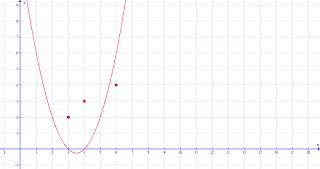## Monday, September 18, 2017

### Find an equation that passes through a set of points (Lagrange polynomial interpolation)

When I was in school, I remember learning about how to find the equation of a line that passes through two points. When I asked how to find the equation of a curve instead I was told that this was not possible, probably because there is an infinite number of curves that pass through a finite set of points (as we'll see below). However I would have been happy to learn about this simple method to produce a polynomial curve that passes through any given set of points. In general this task is called interpolation and the particular interpolating method we shall see here is called Lagrange polynomials.

A polynomial is an equation of the form

$$a_n x^n + a_{n-1} x^{n-1} + \dots + a_1 x + a_0$$

For example $2x^3 + 4x^2 - 3x + 1$ is a polynomial where 2 is $a_3$, 4 is $a_2$, 4 is $a_1$ and 1 is $a_0$. A polynomial plus another polynomial is also a polynomial, for example $(x^2 + 2x) + (3x + 2) = x^2 + 5x + 2$. A polynomial times another polynomial is also a polynomial, for example $(x^2 + 2x)(3x + 2) = 3x^3 + 8x^2 + 4x$.

Now on to polynomial interpolation. Let's start with the simplest case, when you have only one point. Let's say you want a polynomial that passes through (3,2), that is, when $x$ is 3, the polynomial should equal 2. In this case our equation can simply be

$$y = 2$$

that is, the polynomial is just 2. The equation is always 2 regardless of where $x$ is so we have found our polynomial.Graph of equation $y = 2$.

Now on to a more interesting case. Let's say we want to pass through these points:
• (3,2)
• (4,3)
• (6,4)

The trick is to add up a set of polynomials each of which goes through one point. We need a polynomial that equals 2 when $x$ is 3, another polynomial that equals 3 when $x$ is 4, and another polynomial that equals 4 when $x$ is 6. But we have to be careful as we don't want these three polynomials to interfere with each other after being added up together. For example if we can't just do $y = (2) + (3) + (4)$ as the two terms will interfere with each other and the result will not pass through any of the points. Instead we need each polynomial to have a shape such that each one passes through its corresponding point but then passes through 0 in place of the remaining points. The three polynomials we need to add up together are:
• Polynomial corresponding to (3,2): must equal 2 when $x$ is 3 and equal 0 when $x$ is 4 or 6.
• Polynomial corresponding to (4,3): must equal 3 when $x$ is 4 and equal 0 when $x$ is 3 or 6.
• Polynomial corresponding to (6,4): must equal 4 when $x$ is 6 and equal 0 when $x$ is 3 or 4.
Since the polynomials equal 0 where the other points are then they will not interfere with each other where the polynomials pass through their corresponding point.

Let's focus on the zeros first. There is an easy trick to ensure that a polynomial goes through zero at certain values of $x$. If you want your polynomial to be zero when $x$ is 4 or 6, then use $(x-4)(x-6)$. In this polynomial, when $x$ is 4 then the first bracket will equal 0, which makes the whole thing 0, and when $x$ is 6 the second bracket will equal 0 which will also make the whole thing 0. This is what $y = (x-4)(x-6)$, $y = (x-3)(x-6)$ and $y = (x-3)(x-4)$ looks like:Graph of equation $y = (x-4)(x-6)$. Passes through zero when $x$ is 4 or 6.Graph of equation $y = (x-3)(x-6)$. Passes through zero when $x$ is 3 or 6.Graph of equation $y = (x-3)(x-4)$. Passes through zero when $x$ is 3 or 4.

See how each curve passes through zero at every point except one? That exception point will be the corresponding point of each polynomial. Adding these polynomials up will not make them interfere with each other at the x-values of each point. But in order to get the resultant polynomial to pass through the points, we need each separate polynomial to pass through its corresponding point whilst still being zero at every other point. For this we apply another easy trick which is to multiply the polynomials by a number. Multiplying an equation by a number will not change where it is zero. It will change the shape of the curve but the places at which it is zero will not be moved. This is what $(x-4)(x-6)$ looks like after being multiplied by 2, 0.5, and -1:Graph of equation $y = 2(x-4)(x-6)$. Passes through zero when $x$ is 4 or 6.Graph of equation $y = 0.5(x-4)(x-6)$. Passes through zero when $x$ is 4 or 6.Graph of equation $y = -(x-4)(x-6)$. Passes through zero when $x$ is 4 or 6.

So we just need to find the number that when multiplied by each separate polynomial will make it pass through its corresponding point. This number is the y-value of the corresponding point divided by the current value of the polynomial there. What we're doing is first making the polynomial equal 1 at its corresponding point and then multiplying it by whatever number we want it to be. For example if you want to multiply the number 2 by a number that will make the result 3, that number should be $\frac{3}{2}$, that is, $2 \times \frac{3}{2} = 3$.

So what is the current value of $(x-4)(x-6)$ at its corresponding point, (3,2)? It's $(3-4)(3-6) = 3$. So by multiplying $(x-4)(x-6)$ by $\frac{2}{(3-4)(3-6)}$ we can make the polynomial pass through 2, without changing where it is zero. This is what $y = (x-4)(x-6)\frac{2}{(3-4)(3-6)}$, $y = (x-3)(x-6)\frac{3}{(4-3)(4-6)}$ and $y = (x-3)(x-4)\frac{4}{(6-3)(6-4)}$ looks like:Graph of equation $y = (x-4)(x-6)\frac{2}{(3-4)(3-6)}$. Passes through corresponding point (3,2) but through zero when $x$ is 4 or 6.Graph of equation $y = (x-3)(x-6)\frac{3}{(4-3)(4-6)}$. Passes through corresponding point (4,3) but through zero when $x$ is 3 or 6.Graph of equation $y = (x-3)(x-4)\frac{4}{(6-3)(6-4)}$. Passes through corresponding point (6,4) but through zero when $x$ is 3 or 4.

Now we can add them up into
$$y = (x-4)(x-6)\frac{2}{(3-4)(3-6)} + (x-3)(x-6)\frac{3}{(4-3)(4-6)} + (x-3)(x-4)\frac{4}{(6-3)(6-4)}$$
which is simplified into $-\frac{1}{6} x^2 + \frac{13}{6} x - 3$

If we added another point at (10,7) then the polynomial passing through all the points would be
$$y = \frac{2(x-4)(x-6)(x-10)}{(3-4)(3-6)(3-10)} + \frac{3(x-3)(x-6)(x-10)}{(4-3)(4-6)(4-10)} + \frac{4(x-3)(x-4)(x-10)}{(6-3)(6-4)(6-10)} + \frac{7(x-3)(x-4)(x-6)}{(10-3)(10-4)(10-6)}$$

In general, given points $(x_1,y_1), (x_2,y_2), \dots, (x_n,y_n)$, your polynomial will be
$$\sum^{n}_{i=1} y_i \frac{\prod^{n}_{j=1,j\neq i} (x-x_j)}{\prod^{n}_{j=1,j\neq i} (x_i-x_j)}$$
Notice that you cannot have two points with the same x-value or you'll get a division by zero. Notice also how you are not guaranteed to get an extrapolation from your points. The polynomial will completely derail into an upward or downward curve beyond the range of the given points, regardless of any pattern suggested by the points. Finally your equation will get more and more complicated with every new point, even after simplification. If you have $n$ points then you will have up to $n$ terms in your polynomial. It might be simplifiable into less terms, but that is rare.